# It would be very useful to have a theory about computability of functions R" -> R. Given that there Q.2 are an u...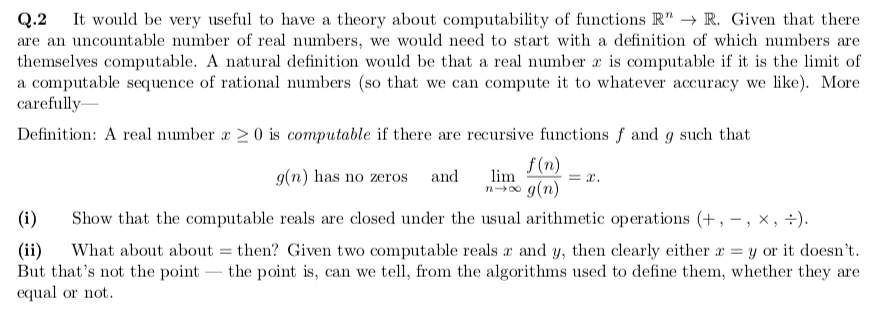It would be very useful to have a theory about computability of functions R" -> R. Given that there Q.2 are an uncountable number of real numbers, we would need to start with a definition of which numbers are themselves computable. A natural definition would be that a real number x is computable if it is the limit of computable sequence of rational numbers (so that we can compute it to whatever accuracy we like). More carefully V a Definition: A real number x 2 0 is computable if there are recursive functions f and g such that f (n) g(n) has no zeros lim and = g(n) Show that the computable reals are closed under the usual arithmetic operations , -, x, -) (i) y or it doesn't (ii) But that's not the point - the point is, can we tell, from the algorithms used to define them, whether they equal or not What about about then? Given two computable reals and y, then clearly either are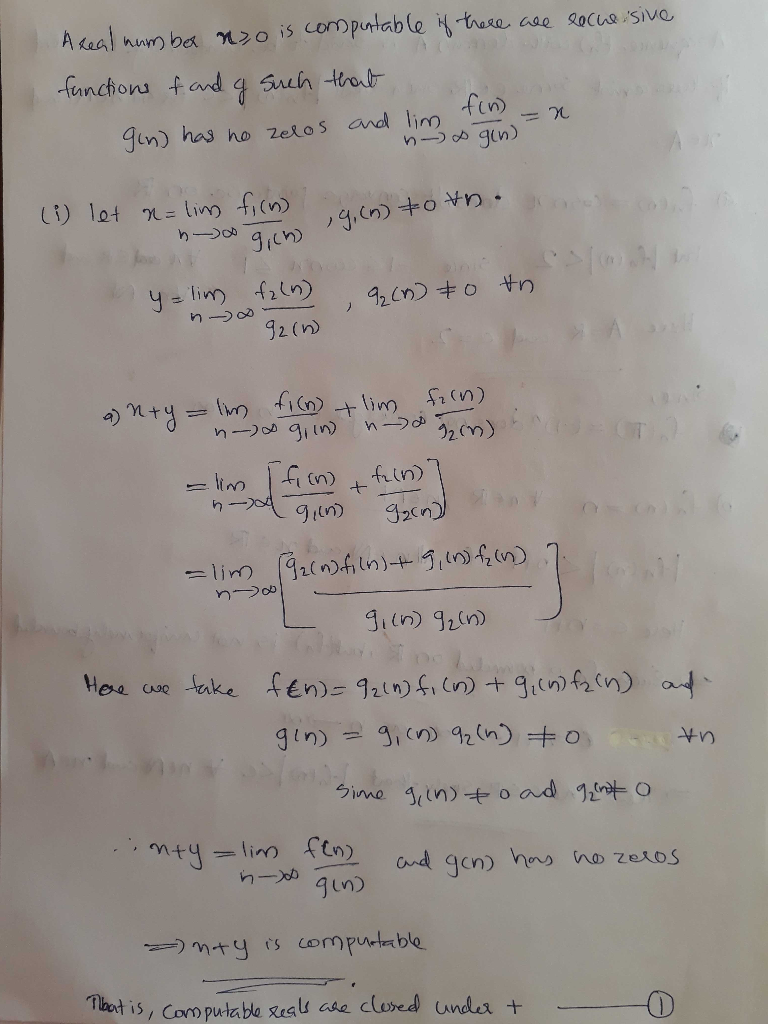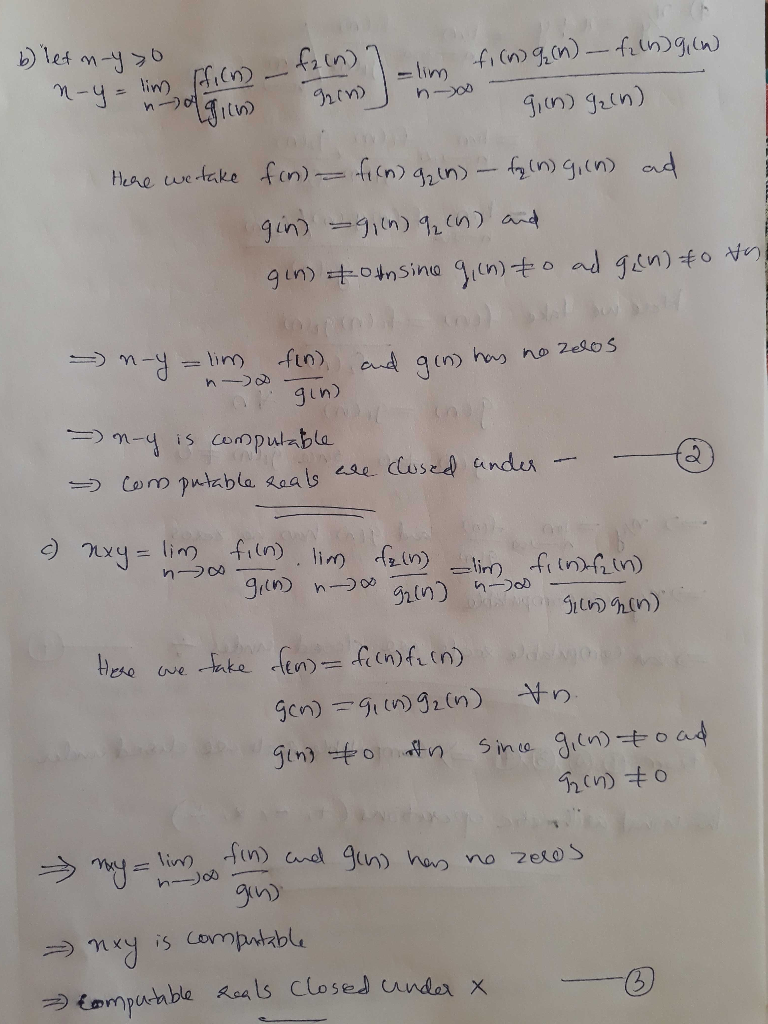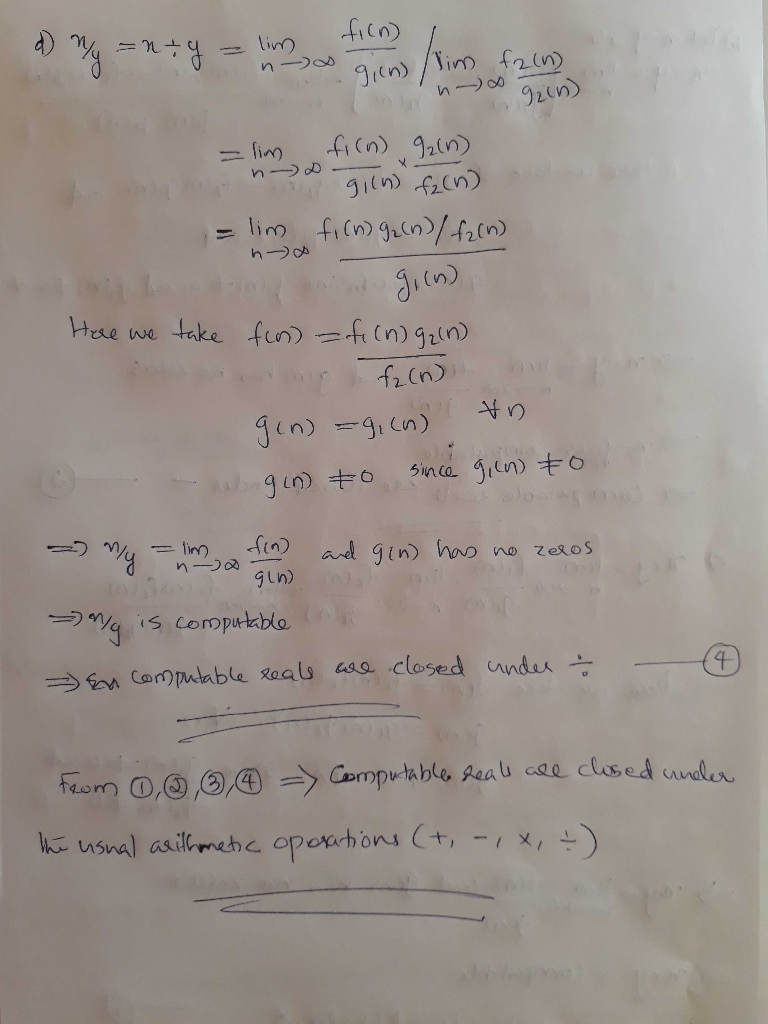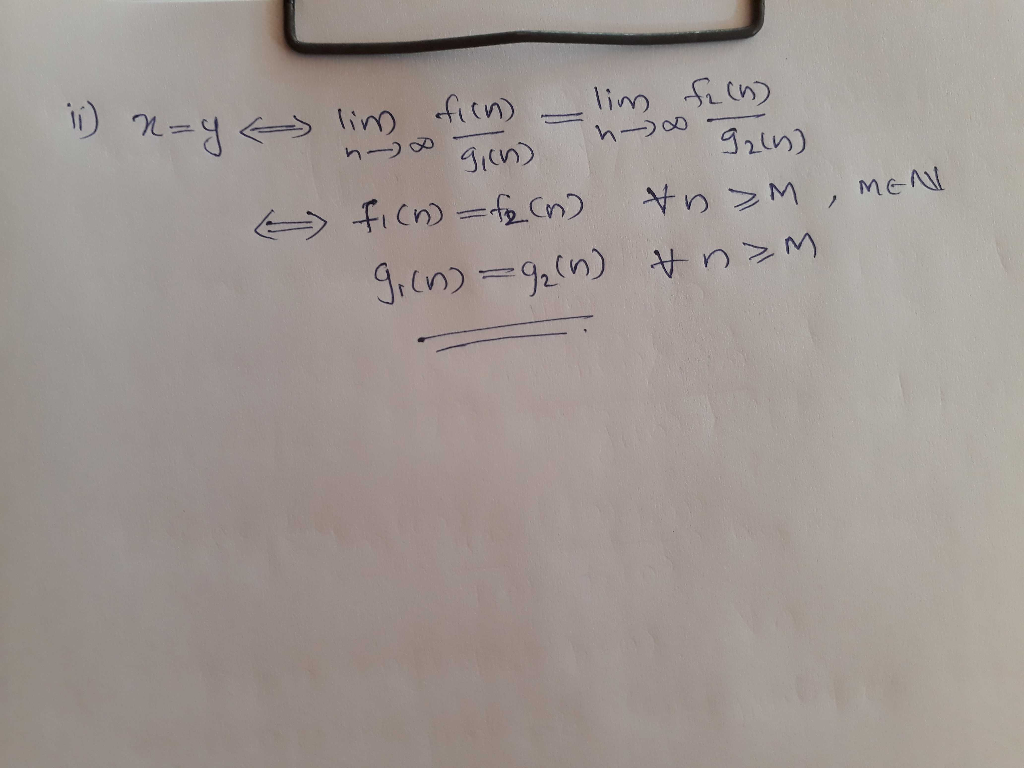##### Add Answer of: It would be very useful to have a theory about computability of functions R" -> R. Given that there Q.2 are an u...
Similar Homework Help Questions
• ### 2. Determine whether the given sets are countable or uncountable. Justify each answer with a bijection...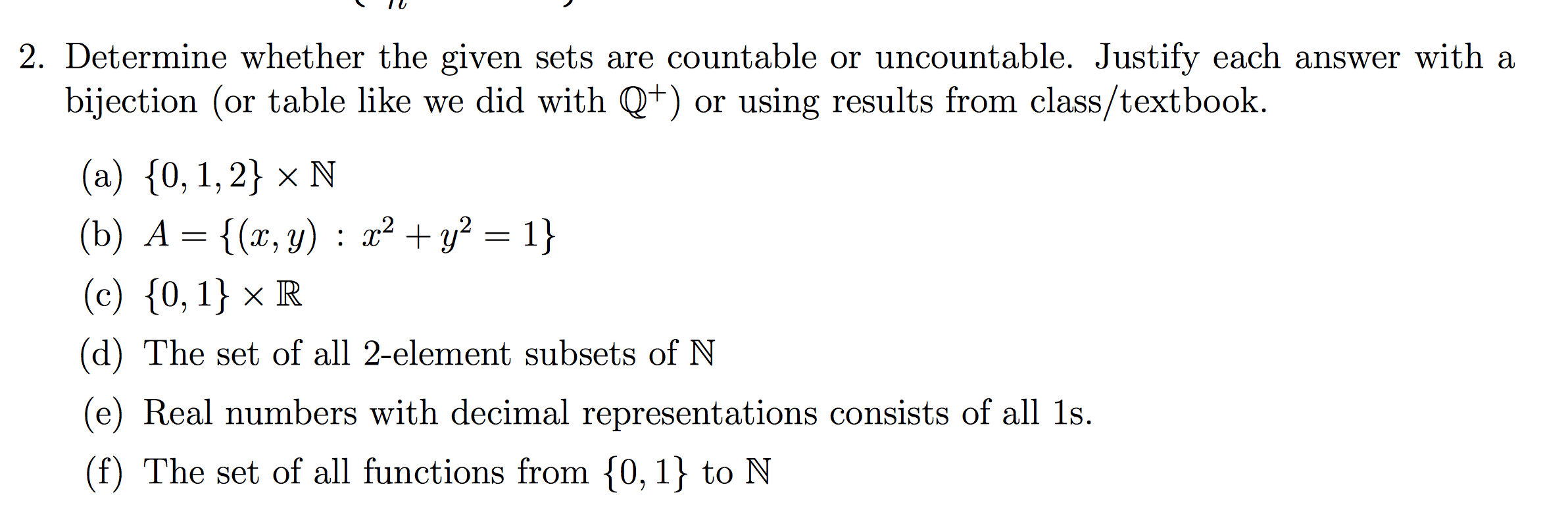2. Determine whether the given sets are countable or uncountable. Justify each answer with a bijection (or table like we did with Q+) or using results from class/textbook. (a) {0, 1, 2} * N (b) A = {(x, y) : x2 + y2 = 1} (c) {0, 1} R Che set of all 2-element subsets of N (e) Real numbers with decimal representations consists of all 1s. (f) The set of all functions from {0,1} to N

• ### In this problem we consider only functions defined on the real numbers R. A function f is close to a function g if 3x E R s.t. Vy E R, A function f visits a function g when Vz E R, R s.t. a<y and...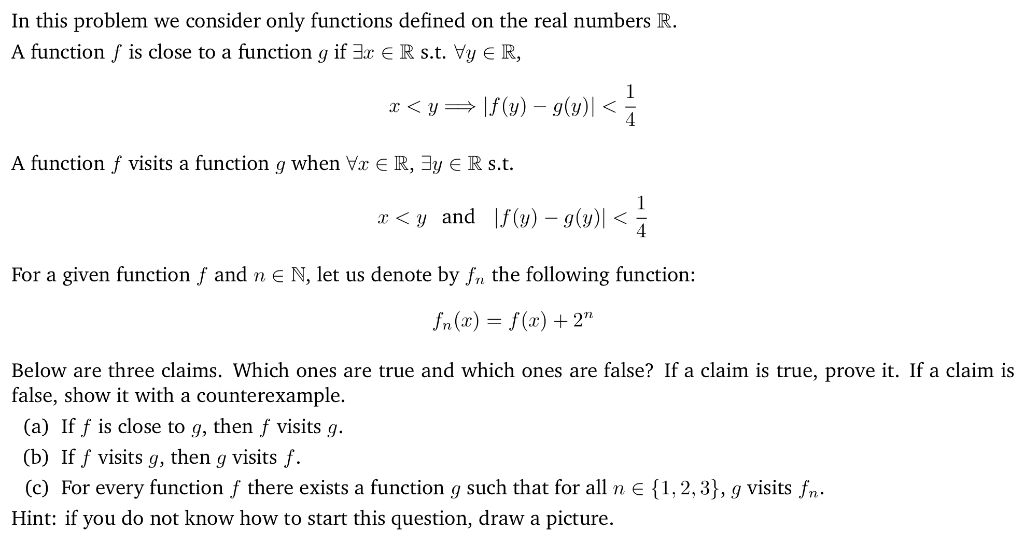In this problem we consider only functions defined on the real numbers R. A function f is close to a function g if 3x E R s.t. Vy E R, A function f visits a function g when Vz E R, R s.t. a<y and f() -g) For a given function f and n E N, let us denote by n the following function: n(x)-f(x)+2" Below are three claims. Which ones are true and which ones are false? If a...

• ### Real analysis 10 11 12 13 please (r 2 4.1 Limit of Function 129 se f: E → R, p is a limit point of E, and li...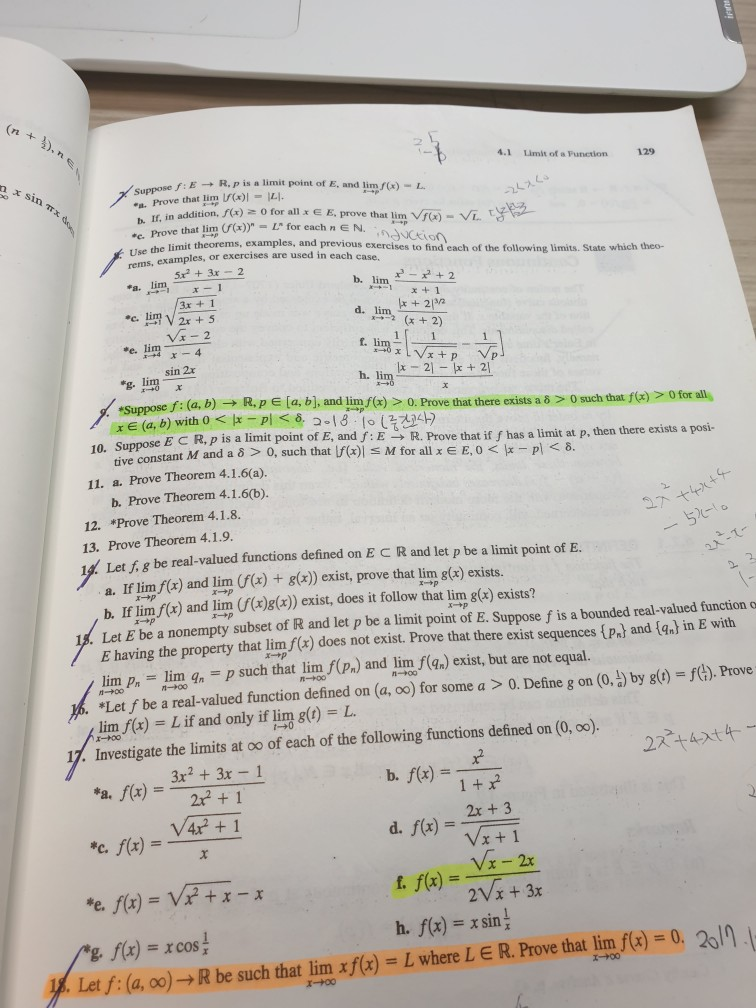Real analysis 10 11 12 13 please (r 2 4.1 Limit of Function 129 se f: E → R, p is a limit point of E, and limf(x)-L. Prove that lim)ILI. h If, in addition, )o for all x E E, prove that lim b. Prove that lim (f(x))"-L" for each n E N. ethe limit theorems, examples, and previous exercises to find each of the following limits. State which theo- rems, examples, or exercises are used in each case....

• ### In this problem we consider only functions defined on the real numbers R. A function f is close to a function g if 3r E R s.t. Vy R, A function f visits a function g when Vz E R,3y E R s.t. < y...

In this problem we consider only functions defined on the real numbers R. A function f is close to a function g if 3r E R s.t. Vy R, A function f visits a function g when Vz E R,3y E R s.t. < y and lf(y)-g(y)| < We were unable to transcribe this imageBelow are three claims. Which ones are true and which ones are false? If a claim is true, prove it. If a claim is false, show...

• ### a) Let f : R → R be a function and CER. Definition 1. The lim+oe an A if for every e>0 there erists a M EN such that for all n 2 M we have lan - A<E Complete the following statement with ou...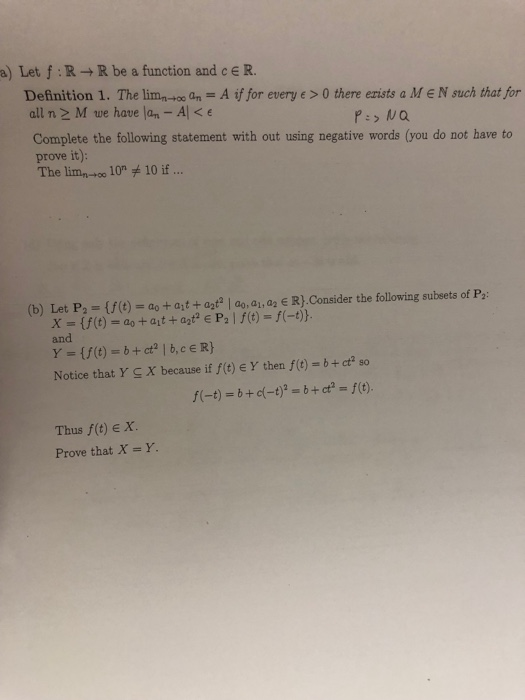a) Let f : R → R be a function and CER. Definition 1. The lim+oe an A if for every e>0 there erists a M EN such that for all n 2 M we have lan - A<E Complete the following statement with out using negative words (you do not have to prove it): The lim, 10 10if R).Consider the following subsets of P: (b) Let P2-(f(t)- ao at + azt | ao, a1, a2 and Notice that Y...

• ### 3. Show that (a) the function g: R” → R, given by g(x) = ||2||2, is...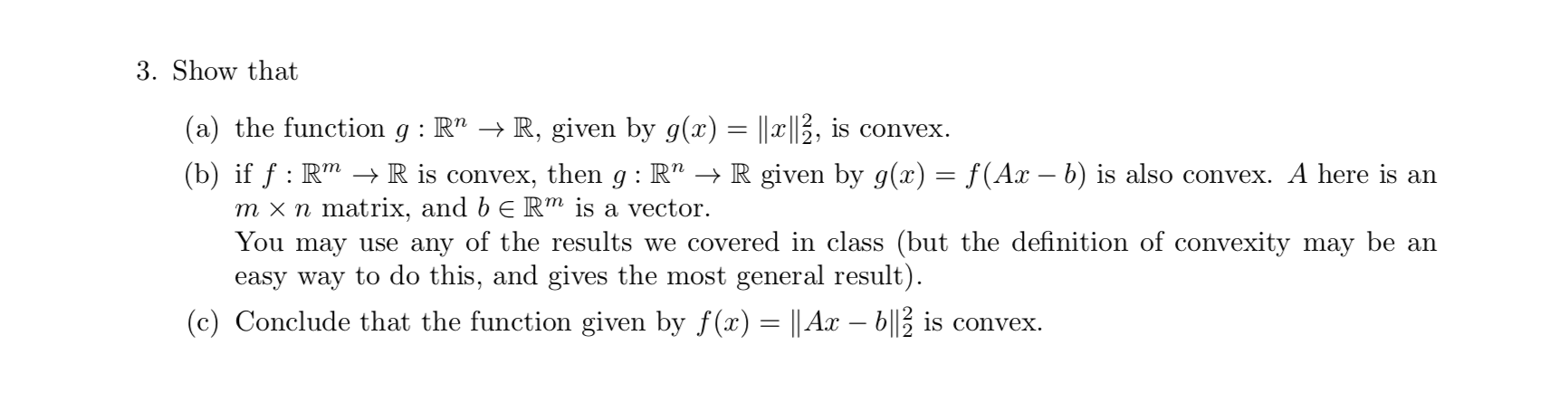3. Show that (a) the function g: R” → R, given by g(x) = ||2||2, is convex. (b) if f : RM → R is convex, then g:R" + R given by g(x) = f(Ax – b) is also convex. A here is an m x n matrix, and b ERM is a vector. You may use any of the results we covered in class (but the definition of convexity may be an easy way to do this, and gives...

• ### plz help me !! Thanks 1. In class we showed that the function f : R → R given by (if>o 0 if a S0 was smooth (but not...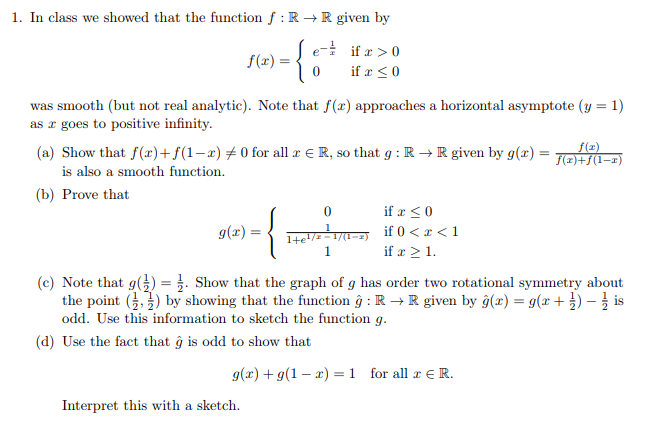plz help me !! Thanks 1. In class we showed that the function f : R → R given by (if>o 0 if a S0 was smooth (but not real analytic). Note that f(x) approaches a horizontal asymptote (y = 1) as a goes to positive infinity. (a) Show that f(x)+f(1-2)メ0 for all x E R, so that g : R → R given by g(x)- 70 is also a smooth function. (b) Prove that if 0 ifx-1. (c) Note...

• ### Discrete Math functions problem - will rate lifesaver!N = set of all non-negative integers, Z = set of all integers, Q = set of all rational numbers, R = set of all real numbers, P = set of allpositive integersWhich of the following are functions from the domain to the codomain given?Which ones are surjective?Which ones areinjective? Which ones are bijective?If they are bijective, describe the inverse function.a) f: Z->N where f is defined by f(x) = -x2 + 2.b) g: P->Q where g is defined by...

• ### Q4 + Fit to page Page view A (1-3)2ary+y'] = x, where y denotes the sum of the given power series with y and y&#...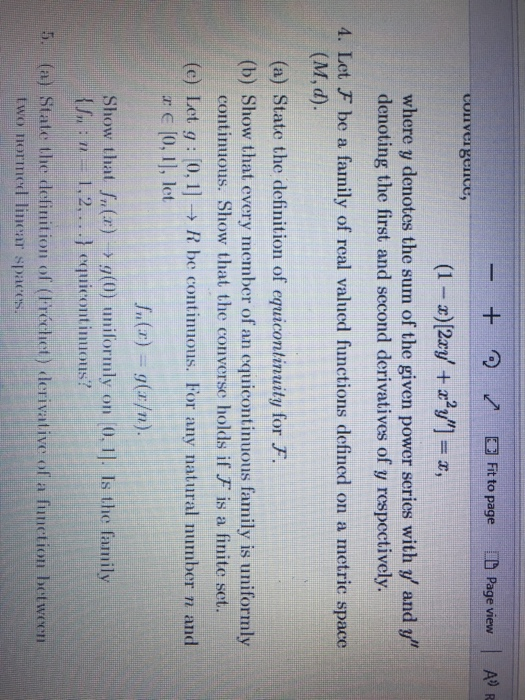Q4 + Fit to page Page view A (1-3)2ary+y'] = x, where y denotes the sum of the given power series with y and y" denoting the first and second derivatives of y respectively 4. Let F be a family of real valued functions defined on a metric space (M, d). (a) State the definition of equicontinuity for F. (b) Show that every member of an cquicontinuous family is uniformly continuous. Show that the converse holds if F is a...

• ### 3. In this problem we consider only functions defined on the real numbers R A function f is close to a function g if r e Rs.t. Vy E R, A function f visits a function g when Vr E R, 3y E R s.t. Fo...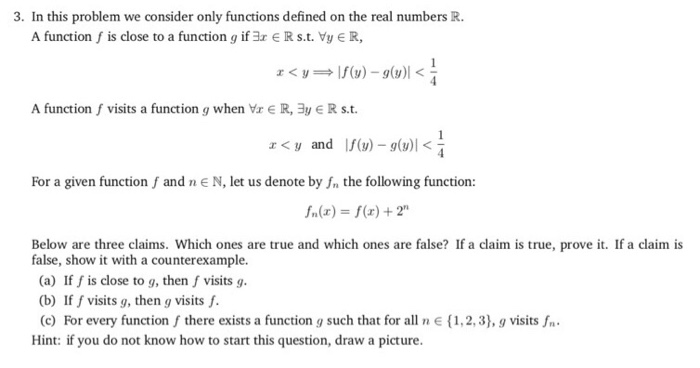3. In this problem we consider only functions defined on the real numbers R A function f is close to a function g if r e Rs.t. Vy E R, A function f visits a function g when Vr E R, 3y E R s.t. For a given function f and n E N, let us denote by fn the following function: Below are three claims. Which ones are true and which ones are false? If a claim is true,...

Need Online Homework Help?